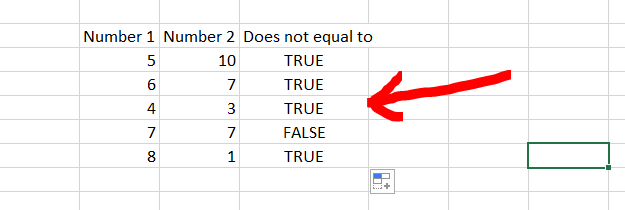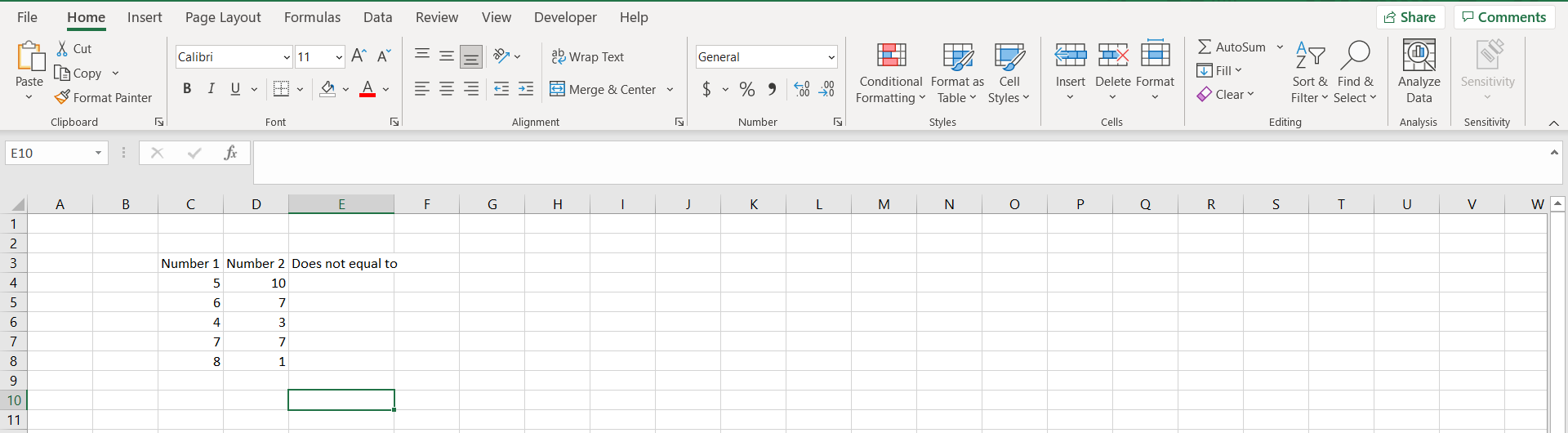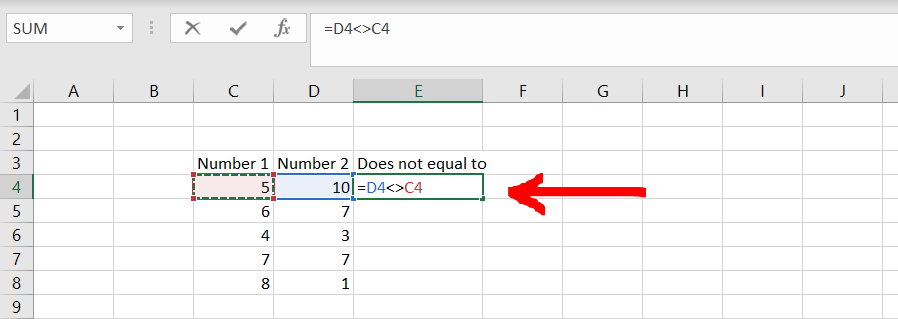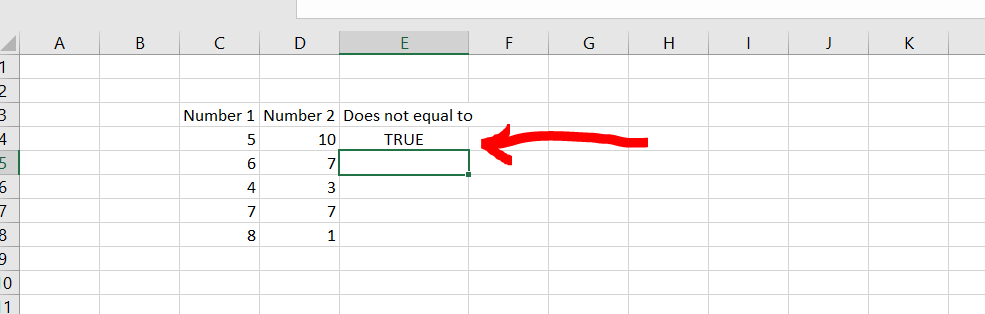# How to write not equal in excelMicrosoft Excel offers a very interesting way to use not equal to operator in excel. We can then perform the below mentioned way to use not equal to operator in excel:

### Step 1 – Excel with some numbers in 2 columns– Open the desired Excel workbook, and make sure there are some numbers in 2 columns to use the not equal to operator.

### Step 2 – Typing the relevant formula– Now as shown in the image above, type the formula “=’Number 1’<>’Number 2’, and hit enter.

### Step 3 – Getting the result– Now since 10 is not equal to 5, we get “TRUE” as our result. We may try to drag the formula for all the rows to get the result for all the rows.

### Step 4 – Getting the result for all the rows– We are able to see that not equal to operator is working fine for the selected range of numbers in our number grid.